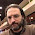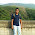## Wednesday, January 05, 2005

### Big Omega

We define big-oh notation by saying f(n)=O(g(n)) if there exists some constant c such that for all large enough n, f(n)≤ c g(n). If the same holds for all c>0, then f(n)=o(g(n)), the little-oh notation. Big-oh and little-oh notation come in very handy in analyzing algorithms because we can ignore implementation issues that could cost a constant factor.

To describe lower bounds we use the big-omega notation f(n)=Ω(g(n)) usually defined by saying for some constant c>0 and all large enough n, f(n)≥c g(n). This has a nice symmetry property, f(n)=O(g(n)) iff g(n)=Ω(f(n)). Unfortunately it does not correspond to how we actually prove lower bounds.

For example consider the following algorithm to solve perfect matching: If the number of vertices is odd then output "No Perfect Matching" otherwise try all possible matchings.

We would like to say the algorithm requires exponential time but in fact you cannot prove a Ω(n2) lower bound using the usual definition of Ω since the algorithm runs in linear time for n odd. We should instead define f(n)=Ω(g(n)) by saying for some constant c>0, f(n)≥ c g(n) for infinitely many n. This gives a nice correspondence between upper and lower bounds: f(n)=Ω(g(n)) iff f(n) is not o(g(n)).

On a related note some researchers like to say f(n)∈O(g(n)) viewing O(g(n)) as a set of functions. This trades off a nice clear unambiguous notation with something ugly for the sake of formality. Yuck.

#### 26 comments:

1.How is f(n) = O(g(n)) nice clear unambiguous?
If f(n) = O(g(n)) and h(n) = O(g(n)), do we have f(n) = h(n)?

(No.)

2.I use the "f(n)=O(g(n))" notation myself, but I must admit that the "f(n) \in O(g(n))" option has merit. First, it is useful to think of this as a set of functions when doing "O arithmetic", as in "(1+o(1))g(n)" or "n^{(O(1))}". Also, it would help the lower bound issues by writing "f(n) \not\in O(g(n))", and saving the Omega notation for the few cases where we need it as it is now.

On the other hand most of us are already used to "f(n)=O(g(n))", and it is not as if as we are anywhere near the level of notational abuse of, say, Quantum Field Theory...

- Eldar.

3.I like the set definition myself, but in writing I might say "T(n) is O(n)" where the "is" is substituting a "=". (Equality is two directional, while "is" is one directional, depending on what the definition of "is" is.)

I'm confused by the litte-oh defintion: isn't f(n)<c*g(n) what it means? (I.e., the same as the big-O defintion, but with a < instead of a >=, and c is always positive?)

4.Usually "f(n)=o(g(n))" means that f(n)/g(n)-->0, or alternatively that for every c>0, |f(n)|<c|g(n)| for n large enough.

- Eldar.

5.I don't understand the statement: "f(n)=?(g(n)) iff g(n) is not o(f(n))"

Consider the case where g(n) represents constant time, and f(n) is say exponential time. Clearly g(n) is a lower bound for f(n), so the condition f(n)=?(g(n)) holds. Also clearly, f(n) is a loose upper bounds for g(n), so g(n) is o(f(n)), contradicting the statement above.

6.I'll try again. (Whatever happened to Unicode?)

I don't understand the statement: "f(n)=Omega(g(n)) iff g(n) is not o(f(n))"

Consider the case where g(n) represents constant time, and f(n) is say exponential time. Clearly g(n) is a lower bound for f(n), so the condition f(n)=Omega(g(n)) holds. Also clearly, f(n) is a loose upper bounds for g(n), so g(n) is o(f(n)), contradicting the statement above.

7.I got that backwards. Should have been "f(n) is Ω(g(n)) iff f(n) is not o(g(n))". I'll fix it in the post.

8.Thanks, makes perfect sense now. -Jon

9.Count one more vote for the "?" crowd. :-) Of course, in keeping with tradition I do use "= O(g(n))" in writing, but I wish everyone would move to the more technically correct "?". The first anonymous commenter has already pointed out my main problem with "=".

-- Amit Chakrabarti

10.Hmm, when I posted the above, I thought I was typing a "belongs to" character (LaTeX $\in$), but it has appeared as "?" on this Firefox window on this Mac.

-AmitC

11.Use &isin; for ∈ and &Omega; for Ω. You can see a list of special characters here.

12.f(n) is O(g(n)) reads better than writing out set membership and is more accurate than using =.

Given this notation there is not need to define the weak Ω at all: Why not simply say that f(n) is not o(g(n)) instead?

The real temptation to use ∈ instead of 'is' comes when doing extensive arithmetic with O(g(n)) quantities.
It is awkward to keep connecting lines with 'which is'
instead of set equality.

13.> We should instead define f(n)=?(g(n)) by saying for some
> constant c>0, f(n)? c g(n) for infinitely many n

This seemed to have been on the cards for some time, atleast according to Knuth's paper, "Big Omicron and Big Omega and Big Theta". He refers to Hardy and Wright preferring "for infinitely many n" instead of "for large n". May be computer scientists did not find it necesary at that time!
amar

14.It is nature that Everybody get confusion with the representation f(n)=O(g(n)). One point I want to clearify is that the above mentioned representation is an assertion. Some author uses this represention(abuse of notation) keeping internally the meaning " f(n) is O(g(n)) or f(n) belongs to O(g(n))".

15.would logn! be big omega of nlogn?

16.If f(x)<=c1O(a(x)) and g(x)<=c2O(b(x))
from comment 1 above

we know that

f(x)*g(x) <= maxof(c1orc2) O(a(x)) * O(b(x))

17.If f(x)<=c1O(a(x)) and g(x)<=c2O(b(x))
from comment 1 above

we know that

f(x)*g(x) <= maxof(c1orc2) O(a(x)) * O(b(x))

18.I have learn discrete math at Univ,
so please show me
how do I choose k for prove Big-O notation ?
what's principle?

19.I have learn discrete math at Univ,
so please show me
how do I choose k for prove Big-O notation ?
what's principle?

20.I have learn discrete math at Univ,
so please show me
how do I choose k for prove Big-O notation ?
what's principle?

21.Is there any positive function f(n) which is neither O(n) nor big-omega(n)??

1.2.n0pe
sara khan

22.nooooooooooooooooooooooooooooooooooooooooooooooooooo

23.in which case omega is required?

24.Yes, Θ(n) which lie between O(n) and big-omega(n),

http://ip-techmania.blogspot.in/2012/06/analysing-big-oh-notation.html